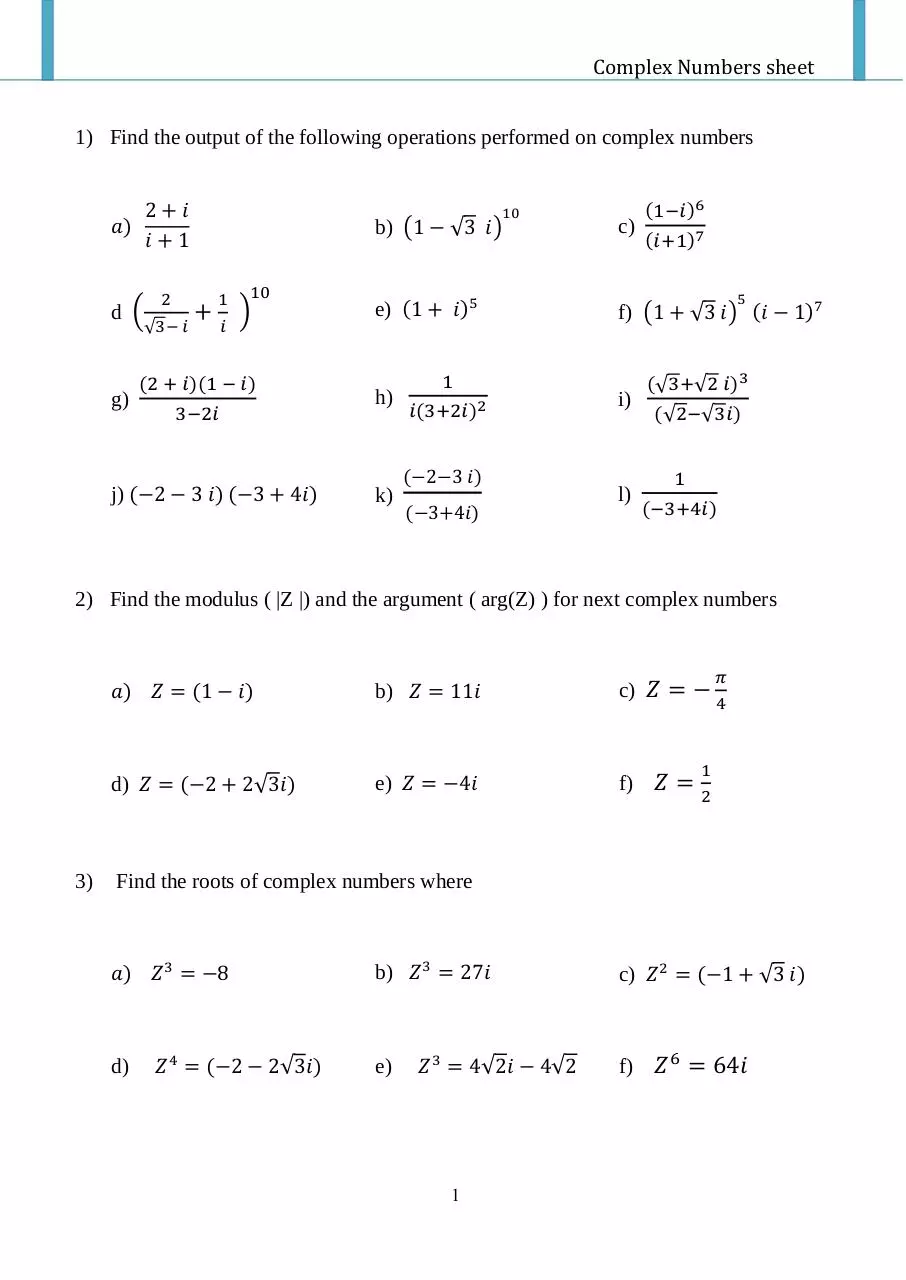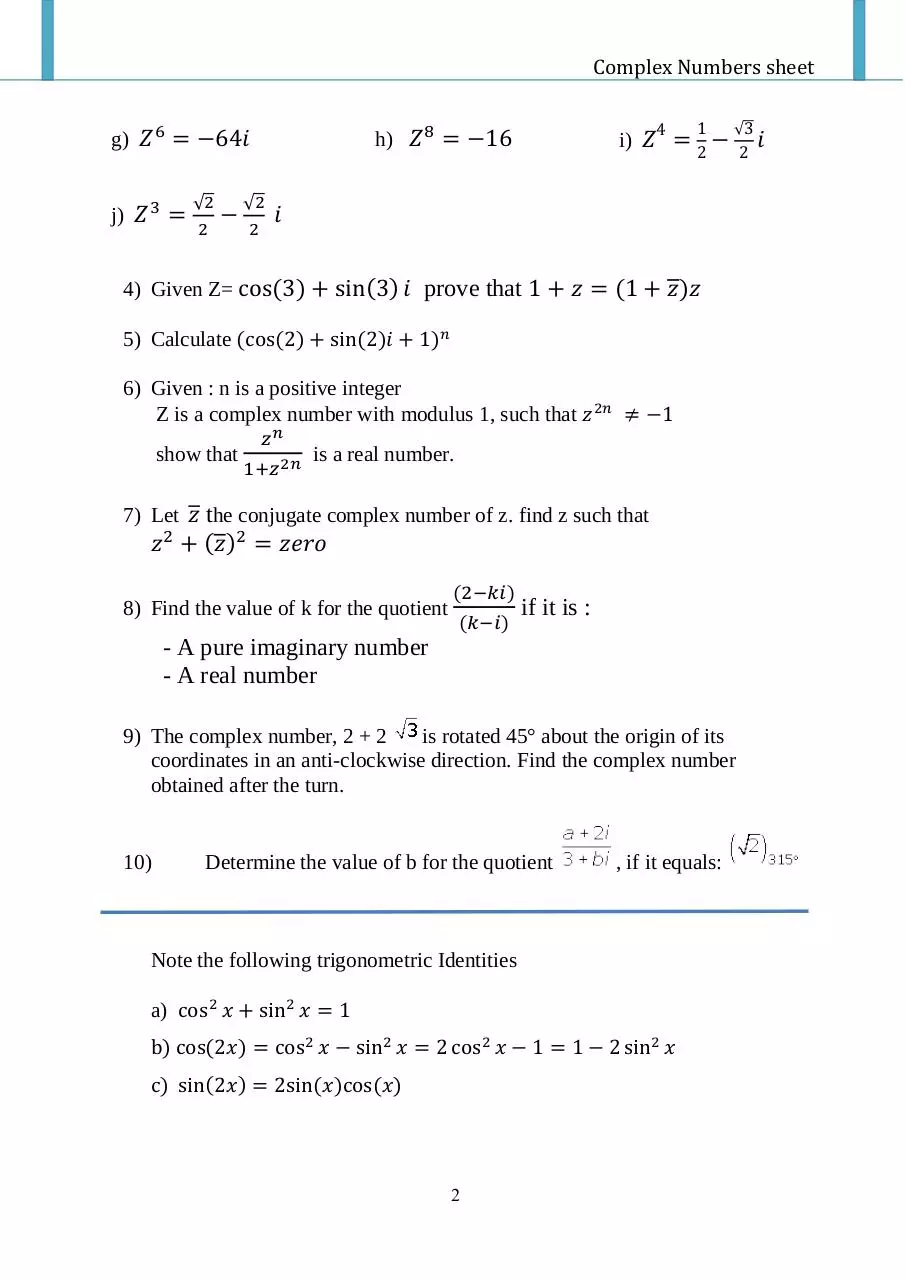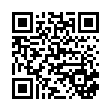# Maths sheet 1 .pdf

### File information

Title: Complex Numbers sheet
Author: mufic

This PDF 1.5 document has been generated by MicrosoftÂ® Word 2013, and has been sent on pdf-archive.com on 06/11/2015 at 09:11, from IP address 41.37.x.x. The current document download page has been viewed 417 times.
File size: 323.86 KB (2 pages).
Privacy: public file

### Document preview

Complex Numbers sheet
1) Find the output of the following operations performed on complex numbers
2+𝑖
𝑎)
𝑖+1
d (

2

√3− 𝑖

g)

10

+

1
𝑖

10

)

(2 + 𝑖)(1 − 𝑖)
3−2𝑖

j) (−2 − 3 𝑖) (−3 + 4𝑖)

(1−𝑖 )6

b) (1 − √3 𝑖)

c)

e) (1 + 𝑖)5

f) (1 + √3 𝑖) (𝑖 − 1)7

h)

1
𝑖(3+2𝑖)2
(−2−3 𝑖)

k)

(−3+4𝑖)

(𝑖+1)7
5

i)

l)

(√3+√2 𝑖)3
(√2−√3𝑖)
1
(−3+4𝑖)

2) Find the modulus ( |Z |) and the argument ( arg(Z) ) for next complex numbers

3)

𝑎) 𝑍 = (1 − 𝑖)

b) 𝑍 = 11𝑖

c) 𝑍 = −

d) 𝑍 = (−2 + 2√3𝑖)

e) 𝑍 = −4𝑖

f) 𝑍 =

𝜋
4

1
2

Find the roots of complex numbers where

𝑎) 𝑍 3 = −8

d)

𝑍 4 = (−2 − 2√3𝑖)

b) 𝑍 3 = 27𝑖

e)

𝑍 3 = 4√2𝑖 − 4√2

1

c) 𝑍 2 = (−1 + √3 𝑖)

f) 𝑍 6 = 64𝑖

Complex Numbers sheet
g) 𝑍 6 = −64𝑖
j) 𝑍 3 =

√2
2

4

h) 𝑍 8 = −16

√2
2

1
2

i) 𝑍 = −

√3

𝑖

4) Given Z= cos(3) + sin(3) 𝑖 prove that 1 + 𝑧 = (1 + 𝑧)𝑧
5) Calculate (cos(2) + sin(2)𝑖 + 1)𝑛
6) Given : n is a positive integer
Z is a complex number with modulus 1, such that 𝑧 2𝑛 ≠ −1
show that

𝑧𝑛

1+𝑧 2𝑛

is a real number.

7) Let 𝑧 the conjugate complex number of z. find z such that

𝑧 2 + (𝑧)2 = 𝑧𝑒𝑟𝑜
8) Find the value of k for the quotient

(2−𝑘𝑖)
(𝑘−𝑖)

if it is :

- A pure imaginary number
- A real number
9) The complex number, 2 + 2
is rotated 45° about the origin of its
coordinates in an anti-clockwise direction. Find the complex number
obtained after the turn.

10)

Determine the value of b for the quotient

, if it equals:

Note the following trigonometric Identities
a) cos 2 𝑥 + sin2 𝑥 = 1
b) cos(2𝑥) = cos 2 𝑥 − sin2 𝑥 = 2 cos 2 𝑥 − 1 = 1 − 2 sin2 𝑥
c) sin(2𝑥) = 2sin(𝑥)cos(𝑥)

2

2

𝑖Maths sheet-1.pdf (PDF, 323.86 KB)

### Share on social networks

#### HTML Code

Copy the following HTML code to share your document on a Website or Blog